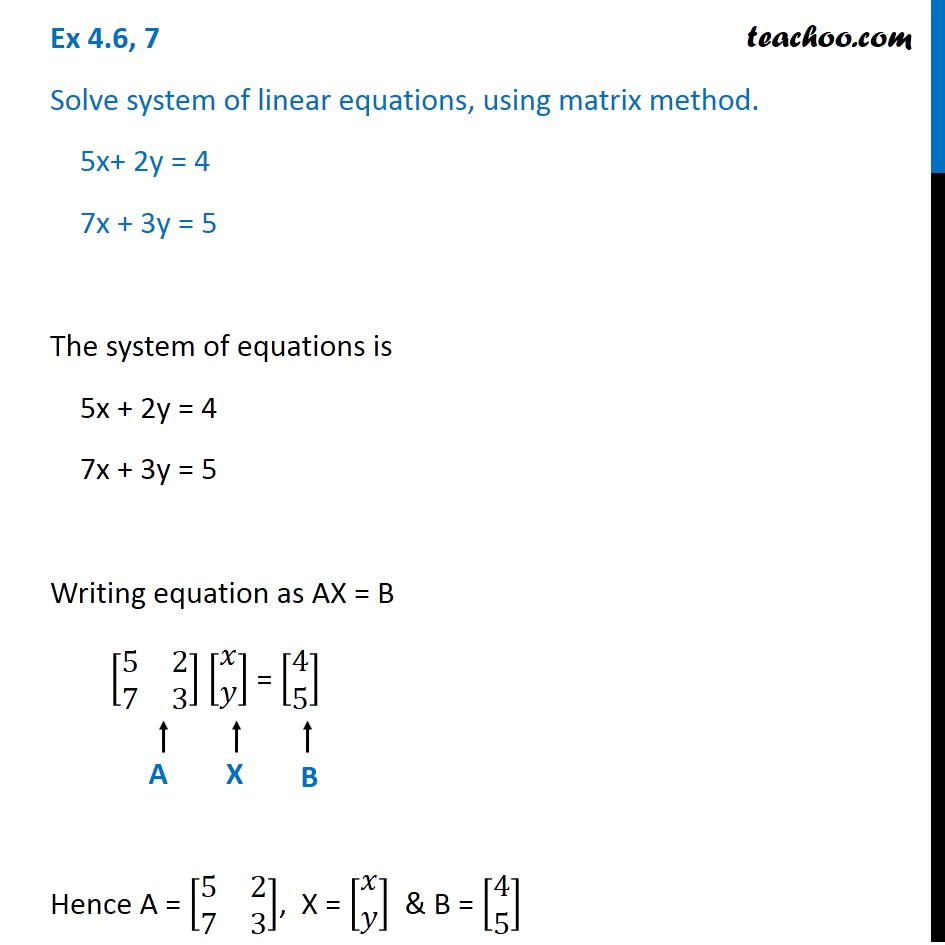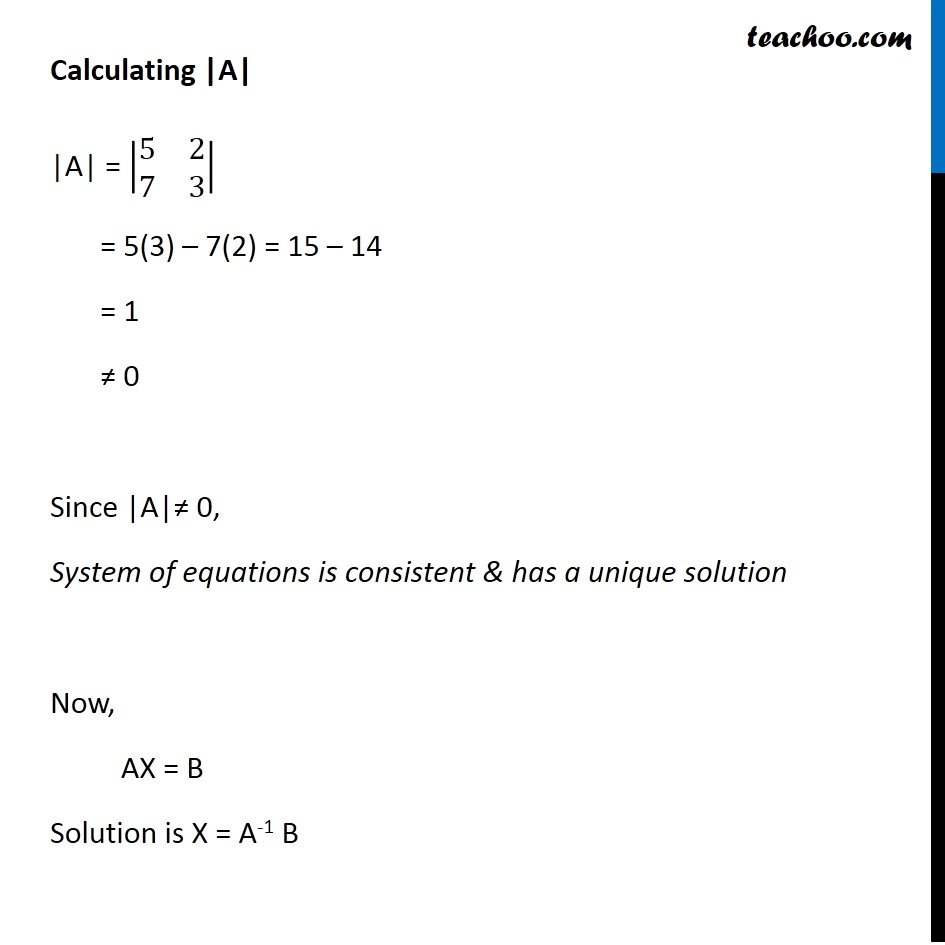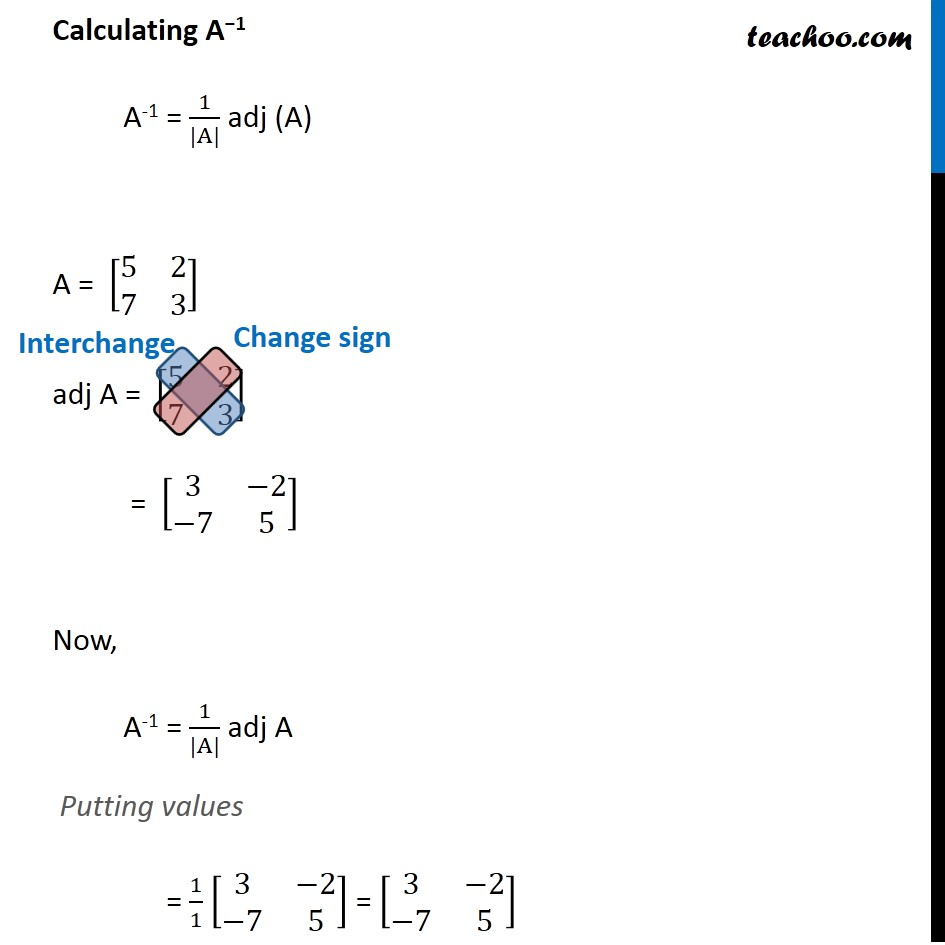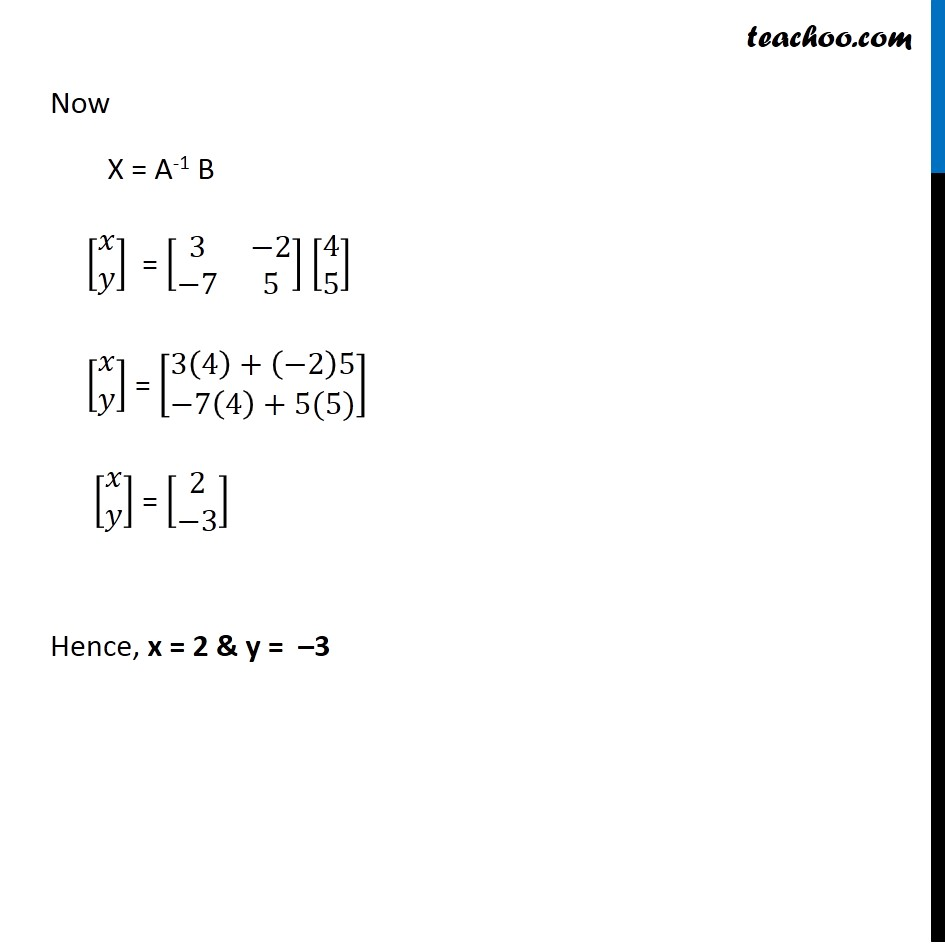Find solution of equations- Equations given

Chapter 4 Class 12 Determinants
Concept wiseLearn in your speed, with individual attention - Teachoo Maths 1-on-1 Class

### Transcript

Ex 4.5, 7 Solve system of linear equations, using matrix method. 5x+ 2y = 4 7x + 3y = 5 The system of equations is 5x + 2y = 4 7x + 3y = 5 Writing equation as AX = B [■8(5&[email protected]&3)] [■8(𝑥@𝑦)] = [■8([email protected])] Hence A = [■8(5&[email protected]&3)], X = [■8(𝑥@𝑦)] & B = [■8([email protected])] Calculating |A| |A| = |■8(5&[email protected]&3)| = 5(3) – 7(2) = 15 – 14 = 1 ≠ 0 Since |A|≠ 0, System of equations is consistent & has a unique solution Now, AX = B Solution is X = A-1 B Calculating A−1 A-1 = 1/(|A|) adj (A) A = [■8(5&[email protected]&3)] adj A = [■8(5&[email protected]&3)] = [■8(3&−2@−7&5)] Now, A-1 = 1/(|A|) adj A Putting values = 1/1 [■8(3&−2@−7&5)] = [■8(3&−2@−7&5)] Now X = A-1 B [■8(𝑥@𝑦)] = [■8(3&−2@−7&5)] [■8([email protected])] [■8(𝑥@𝑦)] = [■8(3(4)+(⤶7−2)5@−7(4)+5(5))] [■8(𝑥@𝑦)] = [■8(2@−3)] Hence, x = 2 & y = –3